# bayesnec

The bayesnec is an R package to fit concentration(dose) — response curves to toxicity data, and derive No-Effect-Concentration (NEC), No-Significant-Effect-Concentration (NSEC), and Effect-Concentration (of specified percentage “x”, ECx) thresholds from non-linear models fitted using Bayesian Hamiltonian Monte Carlo (HMC) via brms (Paul Christian Bürkner 2017; Paul-Christian Bürkner 2018) and stan. The package is an adaptation and extension of an initial package jagsNEC (Fisher, Ricardo, and Fox 2020) which was based on the R2jags package (Su and Yajima 2015) and jags (Plummer 2003).

# Background

Bayesian model fitting can be difficult to automate across a broad range of usage cases, particularly with respect to specifying valid initial values and appropriate priors. This is one reason the use of Bayesian statistics for NEC estimation (or even ECx estimation) is not currently widely adopted across the broader ecotoxicological community, who rarely have access to specialist statistical expertise. The bayesnec package provides an accessible interface specifically for fitting NEC models and other concentration—response models using Bayesian methods. A range of models are specified based on the known distribution of the “concentration” or “dose” variable (the predictor) as well as the “response” variable. The package requires a simplified model formula, which together with the data is used to wrangle more complex non-linear model formula(s), as well as to generate priors and initial values required to call a brms model. While the distribution of the predictor and response variables can be specified directly, bayesnec will automatically attempt to assign the correct distribution to use based on the characteristics of the provided data.

This project started with an implementation of the NEC model based on that described in (Pires et al. 2002) and (Fox 2010) using R2jags (Fisher, Ricardo, and Fox 2020). The package has been further generalised to allow a large range of response variables to be modelled using the appropriate statistical distribution. While the original jagsNEC implementation supported Gaussian-, Poisson-, Binomial-, Gamma-, Negative Binomial- and Beta-distributed response data, bayesnec additionally supports the Beta-Binomial distribution, and can be easily extended to include any of the available brms families. We have since also further added a range of alternative NEC model types, as well as a range of concentration—response models (such as 4-parameter logistic and Weibull models) that are commonly used in frequentist-based packages such as drc(Ritz et al. 2016). These models do not employ segmented linear regression (i.e., use of a step function) but model the response as a smooth function of concentration.

Specific models can be fit directly using bnec, which is what we discuss here. Alternatively, it is possible to fit a custom model set, a specific model set, or all the available models. Further information on fitting multi-models using bayesnec can be found in the Multi model usage vignette. For detailed information on the models available in bayesnec see the Model details vignette.

Important information on the current package is contained in the bayesnec help-files and the Model details vignette.

This package is currently under development. We are keen to receive any feedback regarding usage, and especially bug reporting that includes an easy to run self-contained reproducible example of unexpected behaviour, or example model fits that fail to converge (have poor chain mixing) or yield other errors. Such information will hopefully help us towards building a more robust package. We cannot help troubleshoot issues if an easy-to-run reproducible example is not supplied.

# Installation

To install the latest release version from CRAN use

install.packages("bayesnec")

if (!requireNamespace("remotes")) {
install.packages("remotes")
}
remotes::install_github("open-aims/bayesnec", ref = "dev")

Because bayesnec is based on brms and Stan, a C++ compiler is required. The program Rtools comes with a C++ compiler for Windows. On Mac, you should install Xcode. See the prerequisites section on this link for further instructions on how to get the compilers running.

To run this vignette, we will also need some additional packages

library(dplyr)
library(ggplot2)
library(tidyr)

# Examples

## Fitting the nec4param model using bnec

Here we include some examples showing how to use the package to fit an NEC model to binomial, proportional, count and continuous response data. The examples are those used at: https://github.com/gerard-ricardo/NECs/blob/master/NECs, but here we are showing how to fit models to these data using the bayesnec package. Note however, the default behaviour in bayesnec is to use the "identity" link because the native link functions for each family (e.g., "logit" for Binomial, "log" for Poisson) introduce non-linear transformation on formulas which are already non-linear. So please be aware that estimates of NEC or ECx might not be as expected when using link functions other than identity (see the Model details vignette for more information and the models available in bayesnec).

### Binomial data

The response variable is considered to follow a binomial distribution when it is a count out of a total (such as the percentage survival of individuals, for example). First, we read in the binomial example from pastebin, prepare the data for analysis, and then inspect the dataset as well as the “concentration”, in this case raw_x.

binom_data <- "https://pastebin.com/raw/zfrUha88" |>
dplyr::rename(raw_x = raw.x) |>
dplyr::mutate(raw_x = as.numeric(as.character(raw_x)))

str(binom_data)
#> 'data.frame':    48 obs. of  3 variables:
#>  $raw_x: num 0.1 0.1 0.1 0.1 0.1 0.1 6.25 6.25 6.25 6.25 ... #>$ suc  : int  101 106 102 112 58 158 95 91 93 113 ...
#>  $tot : int 175 112 103 114 69 165 109 92 99 138 ... summary(binom_data$raw_x)
#>    Min. 1st Qu.  Median    Mean 3rd Qu.    Max.
#>    0.10   10.94   37.50   99.23  125.00  400.00

In this case for raw_x, lowest concentration is 0.1 and the highest is 400. The data are right skewed and on the continuous scale. This type of distribution for the predictor data are common for concentration—response experiments, where the concentration data are the concentration of contaminants, or dilutions. The current default in bayesnec is to estimate the appropriate distribution(s) and priors based on the data, but it is possible to supply prior or family directly as additional arguments to the underlying brm function from brms. We are going to model this with the predictor data on a log scale, as this is the scaling clearly used in the experimental design and thus will provide more stable results.

The data are binomial, with the column suc indicating the number of successes in the binomial call, and column tot indicating the number of trials.

The main working function in bayesnec is the function bnec, which calls the other necessary functions and fits the brms model. We can run bnec by supplying formula: a special formula which comprises the relationship between response and predictor, and the C-R model (or models) chosen to be fitted; and data: a data.frame containing the data for the model fitting, here, binom_data.

The basic bayesnec formula should be of the form:

suc | trials(tot) ~ crf(log(raw_x), model = "your_model")

where the left-hand side of the formula is implemented exactly as in brms, including the trials term. The right-hand side of the formula contains the special internal function crf (which stands for concentration-response function), and takes the predictor (including any simple function transformations such as log) and the desired C-R model or suite of models. As with any formula in R, the name of the terms need to be exactly as they are in the input data.frame.

bnec will guess the data types for use, although as mentioned above we could manually specify family as "binomial" (or binomial, or binomial()). bnec will also generate appropriate priors for the brms model call, although these can also be specified manually (see the Priors vignette for more details).

library(bayesnec)

set.seed(333)
exp_1 <- bnec(suc | trials(tot) ~ crf(log(raw_x), model = "nec4param"),
data = binom_data)

The function shows the progress of the brms fit and returns the usual brms output (with a few other elements added to this list). The function plot(pull_brmsfit(exp_1)) can be used to plot the chains, so we can assess mixing and look for other potential issues with the model fit. We can also run a pairs plot that can help to assess issues with identifiability, and which also looks OK. There are a range of other model diagnostics that can be explored for brms model fits, using the function pull_brmsfit. We encourage you to explore the rich material already on GitHub regarding use and validation of brms models.

Initially bayesnec will attempt to use starting values generated for that type of model formula and family. It will run the iterations and then test if all chains are valid. If the model does not have valid chains bayesnec will discard that model and an error will be returned indicating the model could not be fit successfully.

Whenever the model argument of crf is a single model bnec will return an object of class bayesnecfit. We can see the summary of our fitted model parameters using:

summary(exp_1)
#> Object of class bayesnecfit containing the following non-linear model: nec4param
#>
#>  Family: binomial
#> Formula: suc | trials(tot) ~ bot + (top - bot) * exp(-exp(beta) * (log(raw_x) - nec) * step(log(raw_x) - nec))
#>          bot ~ 1
#>          top ~ 1
#>          beta ~ 1
#>          nec ~ 1
#>    Data: data (Number of observations: 48)
#>   Draws: 4 chains, each with iter = 10000; warmup = 8000; thin = 1;
#>          total post-warmup draws = 8000
#>
#> Population-Level Effects:
#>      Estimate Est.Error l-95% CI u-95% CI Rhat Bulk_ESS Tail_ESS
#> bot      0.05      0.02     0.02     0.08 1.00     3260     2641
#> top      0.83      0.01     0.81     0.84 1.00     4225     4343
#> beta     0.81      0.13     0.56     1.08 1.00     2971     3042
#> nec      4.43      0.03     4.36     4.48 1.00     3425     3777
#>
#> Draws were sampled using sample(hmc). For each parameter, Bulk_ESS
#> and Tail_ESS are effective sample size measures, and Rhat is the potential
#> scale reduction factor on split chains (at convergence, Rhat = 1).
#>
#>
#> Bayesian R2 estimates:
#>    Estimate Est.Error Q2.5 Q97.5
#> R2     0.81      0.00 0.80  0.82

Note the Rhat values in this example are one, indicating convergence.

The functions plot (extending base plot method) and autoplot (extending ggplot2 plot method) can be used to plot the fitted model. You can also make your own plot from the data included in the returned object (class bayesnecfit) from the call to bnec. Notice, however, that in this case we log-transformed the predictor raw_x in the input formula. This causes brms to pass these transformations onto Stan’s native code, and therefore estimates of NEC will be on that same scale. So we need to transform them back to the data scale using the additional argument xform.

autoplot(exp_1, xform = exp)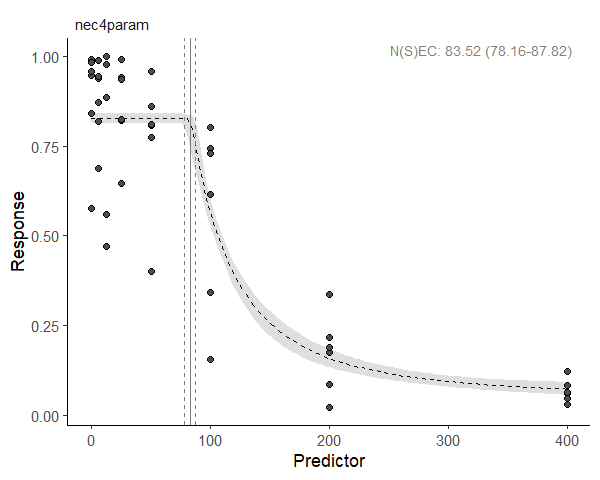There are many built in methods available for brmsfit objects and we encourage you to make use of these in full.

This model fit does not look great. You can see that the error bounds around the fit are far too narrow for this data given the variability among the points, suggesting over dispersion of this model (meaning that the data are more variable than this model fit predicts). For models whose response data are Binomial- or Poisson-distributed, an estimate of dispersion is provided by bayesnec, and this can be extracted using exp_1$dispersion. Values > 1 indicate overdispersion and values < 1 indicate underdispersion. In this case the overdispersion value is much bigger than 1, suggesting extreme overdispersion (meaning our model does not properly capture the true variability represented in this data). We would need to consider alternative ways of modelling this data using a different distribution, such as the Beta-Binomial. exp_1$dispersion
#> Estimate     Q2.5    Q97.5
#> 19.65742 13.22821 30.92392

### Beta-Binomial

The Beta-Binomial model can be useful for overdispersed Binomial data.

set.seed(333)
exp_1b <- bnec(suc | trials(tot) ~ crf(log(raw_x), model = "nec4param"),
data = binom_data, family = beta_binomial2)

Fitting this data with the betabinomial2 yields a much more realistic fit in terms of the confidence bounds (check autoplot(exp_1b, xform = exp)) and the spread in the data. Note that a dispersion estimate is not provided here, as overdispersion is only relevant for Poisson and Binomial data.

autoplot(exp_1b, xform = exp)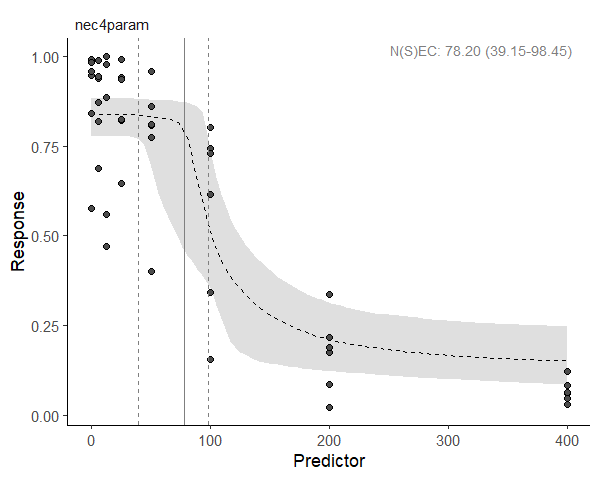exp_1b$dispersion #>  NA NA NA Now that we have a better fit to these data, we can interpret the results. The estimated NEC value can be obtained directly from the fitted model object. As explained above, we need to transform NEC back to the data scale. summary(exp(exp_1b$nec_posterior))
#>    Min. 1st Qu.  Median    Mean 3rd Qu.    Max.
#>   22.16   65.47   78.20   75.93   87.32  198.25

ECx estimates can also be obtained from the NEC model fit, using the function ecx. Note these may differ from a typical 4-parameter non-linear model, as the NEC model is a broken stick non-linear regression and will often fall more sharply than a smooth 4-parameter non-linear curve. See both the Model details and Comparing posterior predictions vignettes for more information.

ecx(exp_1b, xform = exp)
#>      Q50     Q2.5    Q97.5
#> 82.56186 43.33243 98.57387
#> attr(,"precision")
#>  1000
#> attr(,"ecx_val")
#>  10
#> attr(,"toxicity_estimate")
#>  "ecx"

A summary method has also been developed for bayesnecfit objects that gives an overall summary of the model statistics, which also include the estimate for NEC, as the nec_intercept population-level effect in the model—again, its value will depend on the scale of the predictor variable.

summary(exp_1b)
#> Object of class bayesnecfit containing the following non-linear model: nec4param
#>
#>  Family: beta_binomial2
#>   Links: mu = identity; phi = identity
#> Formula: suc | trials(tot) ~ bot + (top - bot) * exp(-exp(beta) * (log(raw_x) - nec) * step(log(raw_x) - nec))
#>          bot ~ 1
#>          top ~ 1
#>          beta ~ 1
#>          nec ~ 1
#>    Data: data (Number of observations: 48)
#>   Draws: 4 chains, each with iter = 10000; warmup = 8000; thin = 1;
#>          total post-warmup draws = 8000
#>
#> Population-Level Effects:
#>      Estimate Est.Error l-95% CI u-95% CI Rhat Bulk_ESS Tail_ESS
#> bot      0.13      0.05     0.03     0.23 1.00     2075     1843
#> top      0.84      0.03     0.78     0.88 1.00     1680     2132
#> beta     0.82      0.65    -0.18     3.09 1.00      718      284
#> nec      4.36      0.19     3.67     4.59 1.00      723      255
#>
#> Family Specific Parameters:
#>     Estimate Est.Error l-95% CI u-95% CI Rhat Bulk_ESS Tail_ESS
#> phi     5.30      1.21     3.31     8.14 1.00     1888     1137
#>
#> Draws were sampled using sample(hmc). For each parameter, Bulk_ESS
#> and Tail_ESS are effective sample size measures, and Rhat is the potential
#> scale reduction factor on split chains (at convergence, Rhat = 1).
#>
#>
#> Bayesian R2 estimates:
#>    Estimate Est.Error Q2.5 Q97.5
#> R2     0.76      0.04 0.67  0.81

### Beta data

Sometimes the response variable is distributed between 0 and 1 but is not a straight-forward Binomial because it is a proportion on the continuous scale. A common example in coral ecology is maximum quantum yield (the proportion of light used for photosynthesis when all reaction centres are open) which is a measure of photosynthetic efficiency calculated from Pulse-Amplitude-Modulation (PAM) data. Here we have a proportion value that is not based on trials and successes. In this case there are no theoretical trials, and the data must be modelled using a Beta distribution instead.

prop_data <- "https://pastebin.com/raw/123jq46d" |>
dplyr::rename(raw_x = raw.x) |>
dplyr::mutate(across(where(is.character), as.numeric))

set.seed(333)
exp_2 <- bnec(resp ~ crf(log(raw_x + 1), model = "nec4param"),
data = prop_data)
autoplot(exp_2, xform = function(x)exp(x) - 1)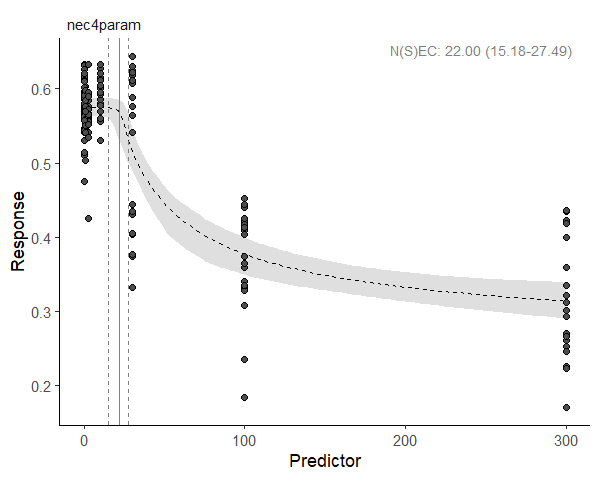### Poisson data

Where data are a count (of, for example, individuals or cells), the response is likely to be Poisson distributed. Such data are distributed from 0 to Inf and are integers. First we mimic count data based on the package’s internal dataset, and make it overdispersed. Then we plot the concentration (i.e. predictor) data.

data(nec_data)
count_data <- nec_data |>
dplyr::mutate(
y = (y * 100 + rnorm(nrow(nec_data), 0, 15)) |>
round() |>
as.integer() |>
abs()
)

str(count_data)
#> 'data.frame':    100 obs. of  2 variables:
#>  $x: num 1.019 0.816 0.371 0.401 1.295 ... #>$ y: int  92 70 87 121 77 66 92 86 108 120 ...
summary(count_data$y) #> Min. 1st Qu. Median Mean 3rd Qu. Max. #> 1.00 67.75 80.50 77.80 95.00 130.00 summary(count_data$x)
#>    Min. 1st Qu.  Median    Mean 3rd Qu.    Max.
#> 0.03235 0.43424 0.87599 1.05142 1.59336 3.22052

First, we supply bnec with data (here count_data), and specify our formula. As we have integers of 0 and greater, the family is "poisson". The default behaviour to guess the variable types works for this example.

set.seed(333)
exp_3 <- bnec(y ~ crf(x, model = "nec4param"), data = count_data)
autoplot(exp_3)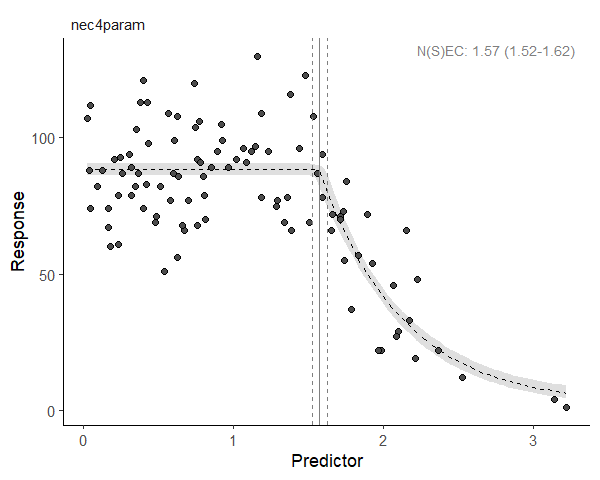exp_3$dispersion #> Estimate Q2.5 Q97.5 #> 4.9198699 0.7354375 153.4861929 We first plot the model chains (with plot(exp_3$fit)) and parameter estimates to check the fit. The chains look OK. However, our plot of the fit is not very convincing. The model uncertainty is very narrow, and this does not seem to be a particularly good model for these data. Note that the dispersion estimate is much greater than one, indicating serious overdispersion. In this case we can try to refit the model but instead deliberately specifying the Negative Binomial distribution for via the family argument.

### Negative binomial

When count data are overdispersed and cannot be modelled using the Poisson distribution, the Negative binomial distribution is generally used. We can do this by calling family = "negbinomial".

set.seed(333)
exp_3b <- bnec(y ~ crf(x, model = "nec4param"), data = count_data,
family = "negbinomial")

The resultant plot seems to indicate the Negative Binomial distribution works better in terms of dispersion (more sensible wider confidence bands), however it still might not be the best model for these data. See the Model details and Multi model usage vignettes for options to fit other models to these data.

autoplot(exp_3b)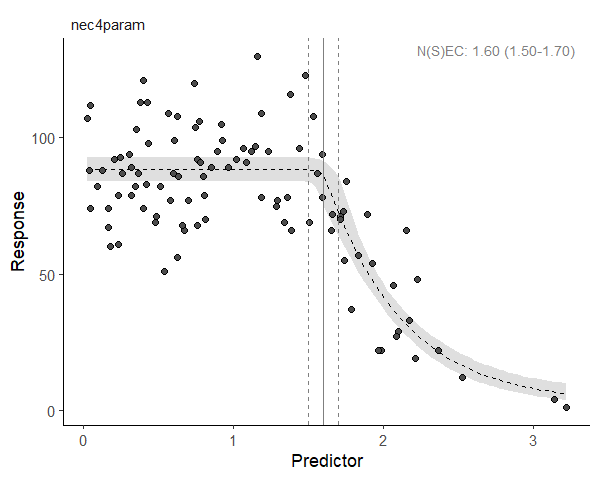### Measure data

Where data are a measured variable, the response is likely to be Gamma distributed. Good examples of Gamma-distributed data include measures of body size, such as length, weight, or area. Such data are distributed from 0+ to Inf and are continuous. Here we use the nec_data supplied with bayesnec with the response y on the exponential scale to ensure the right data range for a Gamma as an example.

data(nec_data)
measure_data <- nec_data |>
dplyr::mutate(measure = exp(y))
set.seed(333)
exp_4 <- bnec(measure ~ crf(x, model = "nec4param"), data = measure_data)
autoplot(exp_4)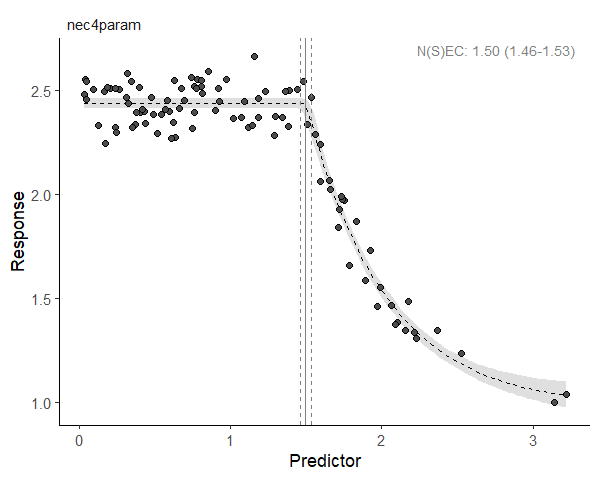summary(exp_4)
#> Object of class bayesnecfit containing the following non-linear model: nec4param
#>
#>  Family: gamma
#>   Links: mu = identity; shape = identity
#> Formula: measure ~ bot + (top - bot) * exp(-exp(beta) * (x - nec) * step(x - nec))
#>          bot ~ 1
#>          top ~ 1
#>          beta ~ 1
#>          nec ~ 1
#>    Data: data (Number of observations: 100)
#>   Draws: 4 chains, each with iter = 10000; warmup = 8000; thin = 1;
#>          total post-warmup draws = 8000
#>
#> Population-Level Effects:
#>      Estimate Est.Error l-95% CI u-95% CI Rhat Bulk_ESS Tail_ESS
#> bot      0.98      0.05     0.89     1.07 1.00     4177     4606
#> top      2.44      0.01     2.41     2.46 1.00     6506     5287
#> beta     0.65      0.10     0.46     0.84 1.00     3537     4013
#> nec      1.50      0.02     1.46     1.53 1.00     4166     4963
#>
#> Family Specific Parameters:
#>       Estimate Est.Error l-95% CI u-95% CI Rhat Bulk_ESS Tail_ESS
#> shape   517.85     75.05   385.62   683.24 1.00     5707     4976
#>
#> Draws were sampled using sample(hmc). For each parameter, Bulk_ESS
#> and Tail_ESS are effective sample size measures, and Rhat is the potential
#> scale reduction factor on split chains (at convergence, Rhat = 1).
#>
#>
#> Bayesian R2 estimates:
#>    Estimate Est.Error Q2.5 Q97.5
#> R2     0.95      0.00 0.94  0.95

In this case our model has converged really well.